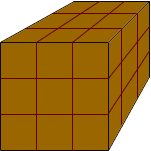Quandaries and Queries My name is Sonya - I am just an Other person, not teacher nor student. � How many cubic feet are in a yard? Thanks. Hi Sonya, The word yard is used in two different senses. In the usual sense a yard is a length. It is 3 feet long or 36 inches long. It is a familiar length as we are accustomed to using yard sticks, which are 36 inches long. Thus it seems strange to compare cubic feet, which measure volume, to yards which measure length. The term yard is, however, used at times to mean cubic yard. If you want a load of topsoil for your garden or concrete to pour a new driveway then the contractor will probably quote you a price in yards. In this situation yards means cubic yards. The lenght one yard is three feet so a cube which is one yard in each direction is three feet by three feet by three feet as in the diagram below.Thus one cubic yard (or yard if you prefer) is 27 cubic feet. Penny Go to Math Central